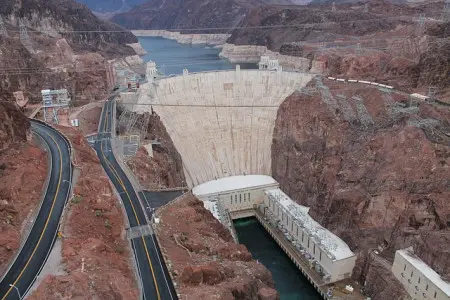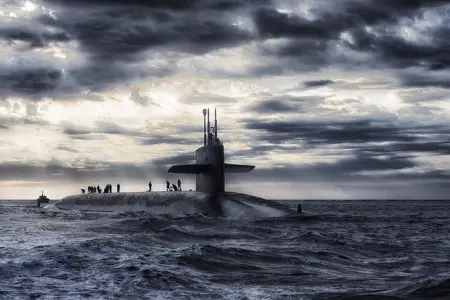# Hydrostatic Pressure: Definition, Formulas, Examples and UsesHydrostatic pressure is a fundamental concept in physics that plays a crucial role in fluid mechanics.

In this article, we will explain in detail what hydrostatic pressure is, how it is calculated using its formula, provide illustrative examples, and discuss its numerous applications in physics, engineering, and everyday life. In addition, we will solve some practical exercises related to hydrostatic pressure to help you better understand this concept.

## What Is Hydrostatic Pressure?

Hydrostatic pressure, also known as rest fluid pressure, refers to the pressure exerted by a fluid at rest due to the column of that fluid above a specific point in it. In other words, when an incompressible fluid, such as a liquid, is at rest, it exerts a downward force on any object immersed in it.This phenomenon is easily observed when we submerge ourselves in water. The deeper we dive, the greater the pressure we feel in our ears and body. This physical property is responsible for this sensation and is the reason why our ears feel clogged when diving in deep water.

## Formula and Calculation

Hydrostatic pressure is calculated using the following formula:

P=ρ⋅g⋅h

Where:

• P is the hydrostatic pressure at the given point (in pascals, Pa).

• ρ is the density of the fluid (in kilograms per cubic meter, kg/m³).

• g is the acceleration due to gravity (approximately 9.81 m/s² at the Earth's surface).

• ℎ is the height of the fluid column above the point of interest (in meters, m).

This equation shows that the hydrostatic pressure is directly proportional to the density of the fluid and the height of the fluid column. The greater the density of the depth at which we are submerged, the greater the hydrostatic pressure.

## Application Examples

Hydrostatic pressure has a wide range of applications in physics, engineering, and everyday life.

Below are some of its most important applications:

### Hydraulics

Hydrostatic pressure is used in hydraulic systems to transmit force and energy.

For example, in heavy machinery, such as excavators, hydraulic presses, and hydraulic elevators, hydrostatic pressure is used along with Pascal's principle to lift heavy objects and perform mechanical work.

### Dam DesignIn civil engineering, hydrostatic pressure plays a crucial role in the design and construction of dams. Dams retain large amounts of water and must withstand the enormous pressures generated by the column of water that accumulates behind them.

In the design of a typical dam we can see how the section at the bottom is thicker than at the top. This is because the pressure exerted by the water increases with increasing depth.

### Medicine

Hydrostatic pressure is essential in blood circulation and organ function. Blood circulates through arteries and veins, and hydrostatic pressure ensures proper flow.

It is also used in the administration of intravenous fluids in medicine, where the speed and height of the fluid bag are adjusted to ensure safe administration.

### Meteorology

Barometers measure atmospheric pressure, which is the pressure exerted by the air column on the Earth's surface. These instruments are essential in meteorology to determine atmospheric conditions and predict the weather.

Variations in atmospheric pressure indicate changes over time, such as the arrival of weather systems or fronts.

## Solved Exercises

Now, let's solve some practical exercises related to hydrostatic pressure:

### Exercise 1

Calculate the pressure at the bottom of a pool 4 meters deep. The density of water is 1000 kg/m³.

Solution

We use the hydrostatic pressure formula:

P=ρ⋅g⋅h

P=(1000kg/m³)⋅(9.81m/s²)⋅(4m)=39240Pa

The pressure at the bottom of the pool is 39,240 pascals.

### Exercise 2A submarine is located at a depth of 500 meters in the ocean, where the density of water is 1030 kg/m³. What is the hydrostatic pressure in the submarine?

Solution

Using the hydrostatic pressure formula:

P=ρ⋅g⋅h

P=(1030 kg/m³)⋅(9.81m/s²)⋅(500m)=5083650Pa

The hydrostatic pressure in the submarine at 500 meters depth is 5,083,650 pascals.

## Conclusion

Hydrostatic pressure is a fundamental concept in physics that is based on the idea that a fluid at rest exerts downward pressure due to the column of fluid above a specific point.

Its formula and illustrative examples demonstrate how it is calculated and how it affects objects immersed in a fluid. In addition, we have explored various applications in engineering, medicine and meteorology, highlighting its importance in our daily lives.

Finally, the solved exercises provided help reinforce the understanding of this fundamental concept in physics.

Author:

Published: September 22, 2023
Last review: September 22, 2023